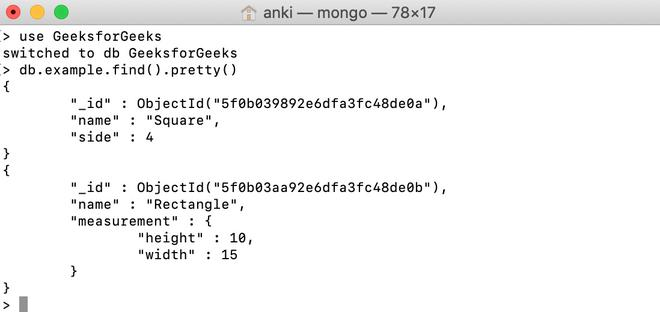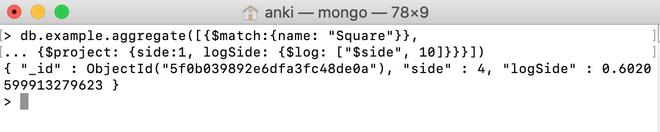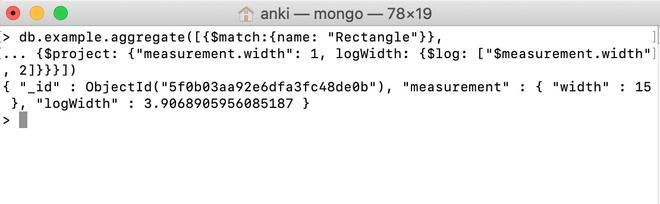Skip to content
Related Articles
MongoDB \$log Operator
• Last Updated : 28 Jul, 2020

MongoDB provides different types of arithmetic expression operators that are used in the aggregation pipeline stages and \$log operator is one of them. This operator is used to find the log of a number in the specified base and returns the result as a double.

Syntax:

```{ \$log: [ <number>, <base> ] }
```

Here, the number is a valid expression as long as it resolves to a non-negative number and base is a valid expression until it resolves to a positive number greater than 1.

• If the entered value is null, then this operator will return null.
• If the entered value is NaN, then this operator will return NaN.
• If the entered value is a missing field, then this operator will return null.

Examples:

In the following examples, we are working with:

Database: GeeksforGeeks

Collection: example

Document: two documents that contain the details of the shapes in the form of field-value pairs.Using \$log operator:

In this example, we are going to find the log of the value of the side field in the square document. Here, the base value is 10.

```db.example.aggregate([{\$match:{name: "Square"}},
... {\$project: {side:1, logSide: {\$log: ["\$side", 10]}}}])
```Using \$log operator in the embedded document:

In this example, we are going to find the log of the value of the measurement.width field in the rectangle document. Here, the base value is 2.

```db.example.aggregate([{\$match:{name: "Rectangle"}},
... {\$project: {"measurement.width": 1,
logWidth: {\$log: ["\$measurement.width", 2]}}}])
```My Personal Notes arrow_drop_up